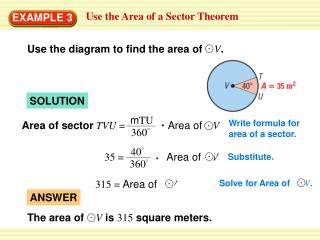DownloadDownload PresentationEXAMPLE 3

# EXAMPLE 3

Télécharger la présentation## EXAMPLE 3

- - - - - - - - - - - - - - - - - - - - - - - - - - - E N D - - - - - - - - - - - - - - - - - - - - - - - - - - -
##### Presentation Transcript

1. 315 = Area ofV Solve for Area of V. The area of Vis 315 square meters. mTU Area of sectorTVU = Area ofV 360° 40° 35 = Area ofV ANSWER 360° Use the Area of a Sector Theorem EXAMPLE 3 Use the diagram to find the area of V. SOLUTION Write formula for area of a sector. Substitute.

2. Standardized Test Practice EXAMPLE 4 SOLUTION The area you need to paint is the area of the rectangle minus the area of the entrance. The entrance can be divided into a semicircle and a square.

3. 180° = 36(26) – (π 82 ) + 162 360° ANSWER The correct answer is C. Standardized Test Practice EXAMPLE 4 = 936 – [32π+ 256] ≈ 579.47 The area is about 579square feet.

4. 4. Find the area of H. 907.92 = Area ofH Solve for Area of H. ANSWER The area of His 907.92 cm2. mFG Area of sector FHG = Area ofH 360 85 214.37 = Area ofH 360 for Examples 3 and 4 GUIDED PRACTICE SOLUTION Write formula for area of a sector. Substitute.

5. 5. Find the area of the figure. for Examples 3 and 4 GUIDED PRACTICE

6. 1 A = b h 2 1 = 7 7 2 for Examples 3 and 4 GUIDED PRACTICE SOLUTION Take the topas base, which is 7 m and find the area of the triangle STEP 1 Write formula for area of a triangle. Substitute. = 24.5 = 24.5 m

7. 1 A = π r2 2 ANSWER 1 = π (3.5)2 The area of the figure is about 43.74 m2. 2 for Examples 3 and 4 GUIDED PRACTICE STEP 2 find the area of the semicircle Write formula for area of a sector. Substitute. = 19.5 multiply STEP 3 Add the areas Area of figure = Area of triangle + Area of semicircle = 24.5 + 19.5 = 43.74 m2

8. m π r2 A= and if you solve this for m, you get 360 360A π r2 for Examples 3 and 4 GUIDED PRACTICE 6. If you know the area and radius of a sector of a circle, can you find the measure of the intercepted arc? Explain. SOLUTION yes; the formula for the area of sector is